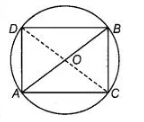# On a common hypotenuse AB,`
Question:

On a common hypotenuse AB, two right angled triangles, ACB and ADB are situated on opposite sides. Prove that ∠BAC = ∠BDC.

Solution:

Given, $\triangle A C B$ and $\triangle A D B$ are two right angled triangles with common hypotenuse $A B$.

To prove $\angle B A C=\angle B D C$

Construction Join $C D$.

Proof Let $O$ be the mid-point of $A B$.

Then, $O A=O B=O C=O D$.Since, mid-point of the hypotenuse of a right triangle is equidistant

from its vertices. Now, draw a circle to pass through the points $A, B, C$ and $D$. with $O$ as centre and radius equal to $O A$.

and radius equal to $O A$.

We know that, angles in the same segment of a circle are equal. From the figure, $\angle B A C$ and $\angle B D C$ are angles of same segment $B C$.

$\therefore \quad \angle B A C=\angle B D C$

Hence proved.